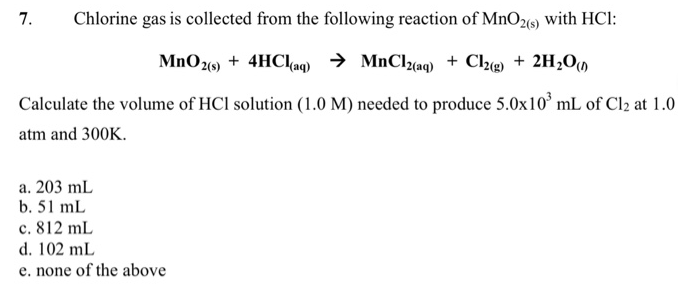# Problem: Chlorine gas is collected from the following reaction of MnO2(s) with HCl:MnO2(s) + 4HCl(aq) → MnCl2(aq) + Cl2(g) + 2H2O(l) Calculate the volume of HCl solution (1.0 M) needed to produce 5.0 x 103 mL of Cl2 at 1.0 atm and 300K. a. 203 mL b. 51 mL c. 812 mL d. 102 mL e. none of the above

###### FREE Expert Solution
84% (26 ratings)###### Problem Details

Chlorine gas is collected from the following reaction of MnO2(s) with HCl:

MnO2(s) + 4HCl(aq) → MnCl2(aq) + Cl2(g) + 2H2O(l)

Calculate the volume of HCl solution (1.0 M) needed to produce 5.0 x 103 mL of Cl2 at 1.0 atm and 300K.

a. 203 mL

b. 51 mL

c. 812 mL

d. 102 mL

e. none of the above